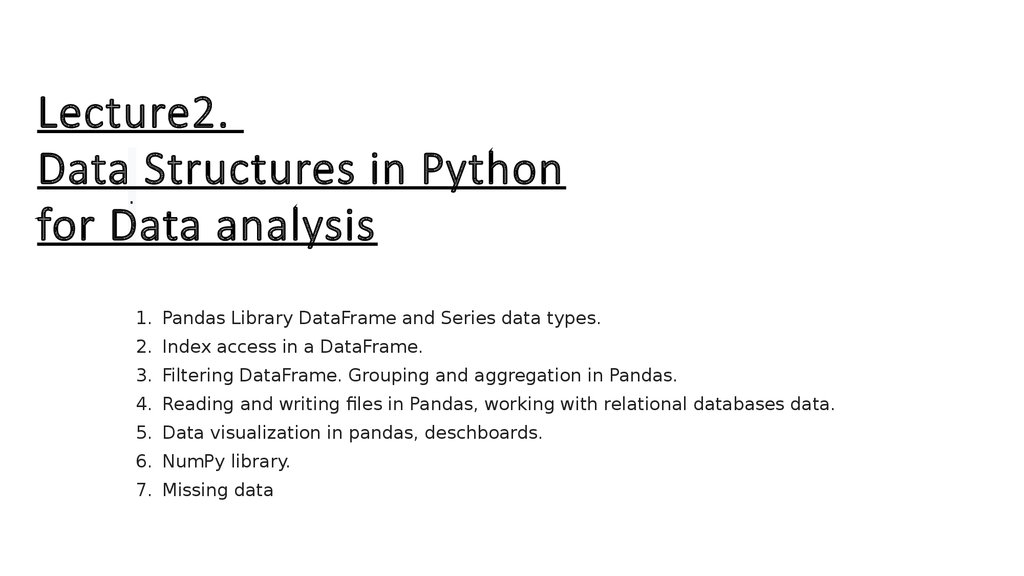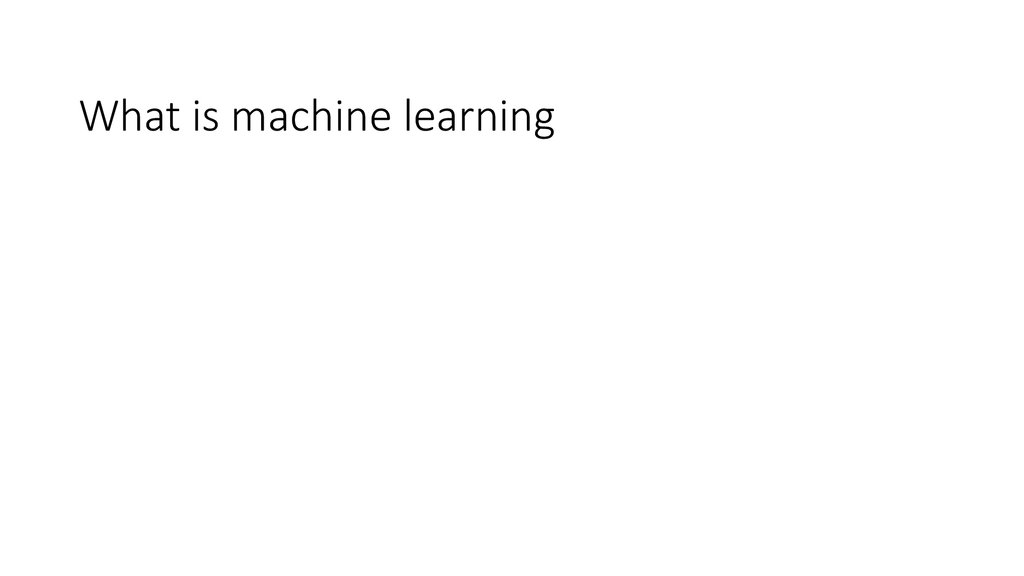# Data. Structures in Python for Data analysis. lecture 2

## 1. .

Lecture2.
Data. Structures in Python
for Data analysis
1. Pandas Library DataFrame and Series data types.
2. Index access in a DataFrame.
3. Filtering DataFrame. Grouping and aggregation in Pandas.
4. Reading and writing files in Pandas, working with relational databases data.
5. Data visualization in pandas, deschboards.
6. NumPy library.
7. Missing data

## 9.

• Machine learning is very useful when no
algorithmic solution is known.
• It also avoids a detailed algorithm to overfit
known cases, reducing classification errors

## 10. What is the goal of machine learning ?

“To build computer systems that
automatically
improve with experience”
Tom M. Mitchell, The discipline of Machine Learning, 2006

## 11. What is machine learning today?

It is mostly learning from (big) data for recognizing patterns

## 12. Python libraries that are useful for developing machine learning solutions

• numpy - a powerful library for scientific computing, particularly for handling N-dimensional
arrays and performing linear algebra operations. Most of your data will be formated using
numpy. Numpy contains core routines for doing fast vector, matrix, and linear algebra-type
operations in Python.
• Scipy contains additional routines for optimization, special functions, and so on. Both
contain modules written in C and Fortran so that they're as fast as possible.
• matplotlib - adds Matlab-like capabilities to Python, including visualization/plotting of data
and images. Useful for inspecting data sets and visualizing results.
• sklearn - a very popular machine learning toolkit for Python with implementations of
almost all common machine learning algorithms and extensions
• Implement decision trees in scikit-learn
• Visualize the decision surface and performance of learned models

## 14. What is a data structure?

• Way to store data and have some method to retrieve and manipulate
it
Lots of examples in python:
• List, dict, tuple, set, string
• Array • Series, DataFrame
• Some of these are “built-in” (meaning you can just use them), others
are contained within other python packages, like numpy and pandas

## 15. Basic Python Data Structures (built-in)

List, dict, tuple, set, string
• Each of these can be accessed in a variety of ways
• Decision on which to use?
Depends on what sort of features you need (easy indexing,
immutability, etc)

## 16. Basic Structure: List

Very versatile, can have items of different types, is mutable
• To create: use square brackets [] to contain comma separated values
• Example:
>> I = ["a", "b", 123]
>> l [’a’, ‘b’, 123]
• To get values out:
>> l
>> b
(use index, starts with 0)

## 17. Basic Structure: Set

Set is an unordered collection with no duplicate values, is mutable
Create using {}
Example:
>> s = {1, 2, 3}
>> s
set([1,2,3])
• Useful for eliminating duplicate values from a list, doing operations
like intersection, difference, union

## 18. Basic Structure: Tuple

Tuple holds values separated by commas, are immutable
• Create using , or () to create empty
Example:
>> t = 1,2,3
>> t (1,2,3)
>> type(t) type ‘tuple’
• Useful when storing data that does not change, when needing to optimize
performance of code (python knows how much memory needed)

## 19. Basic Structure: Dict

Represented by key:value pair
Keys: can by any immutable type and unique
Values: can be any type (mutable or immutable)
To create: use curly braces {} or dict() and list both key and value
>>> letters = {1: ' a', 2: 'b', 3: ' c', 4: 'd'}
>>> type(letters)
To access data in dictionary, call by the key
>>> letters 'b'
Have useful methods like keys(),values(),iteritems(),itervalues() useful for accessing dictionary entries
• Useful when:
• Need association between key:value pair
• Need to quickly look up data based on a defined key
• Values are modified

## 20. Array: Use NumPy!

• What is an array? - “list of lists”
What is NumPy?
• Numerical Python
• Python library very useful for scientific computing
How to access NumPy?
• Need to import it into your python workspace or into your
script
>> import numpy as np

## 29. Pandas: Series

• Effectively a 1-D NumPy array with an index
1D labeled array that can hold any data type, with labels known as the “index”
data can be an array, scalar, or a dict

## 31.

Most commonly used pandas object • DataFrame is basically a
or table of some kind • Can take data from • Dict of 1D arrays,
lists, dicts, Series • 2D numpy array • Series • Another
DataFrame • Can also define index (row labels) and columns
(column labels) • Series can be dynamically added to or
removed from the DataFrame

## 39.

Matplotlib is an excellent 2D and 3D graphics library for generating scientific figures.
Some of the many advantages of this library include:
•Easy to get started
•Support for LATEXLATEX formatted labels and texts
•Great control of every element in a figure, including figure size and DPI.
•High-quality output in many formats, including PNG, PDF, SVG, EPS, and PGF.
•GUI for interactively exploring figures and support for headless generation of figure
files (useful for batch jobs).
One of the key features of matplotlib that I would like to emphasize, and that I think
makes matplotlib highly suitable for generating figures for scientific publications is
that all aspects of the figure can be controlled programmatically. This is important for
reproducibility and convenient
To get started using Matplotlib in a Python program, either include the symbols from
the pylab module (the easy way):